# COP 4710 Database Systems Spring 2004 Day 10

• Slides: 17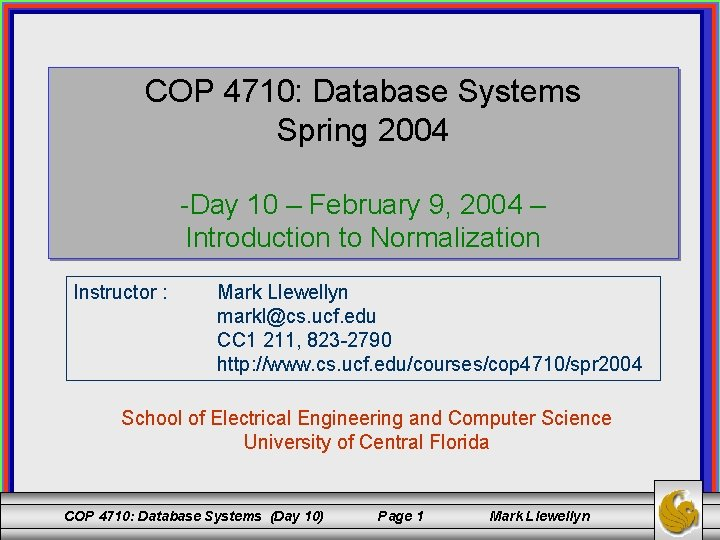COP 4710: Database Systems Spring 2004 -Day 10 – February 9, 2004 – Introduction to Normalization Instructor : Mark Llewellyn [email protected] ucf. edu CC 1 211, 823 -2790 http: //www. cs. ucf. edu/courses/cop 4710/spr 2004 School of Electrical Engineering and Computer Science University of Central Florida COP 4710: Database Systems (Day 10) Page 1 Mark LlewellynProof For Practice Problem in Day 9 Notes • Given R = (A, B, C, D, E, F, G, H, I, J) and F = {AB E, AG J, BE I, E G, GI H} does F ⊨ BE H? Proof 1. 2. 3. 4. 5. 6. 7. 8. BE I, given in F BE BE, reflexive rule IR 1 BE E, projective rule IR 4 from step 2 E G, given BE G, transitive rule IR 3 from steps 3 and 4 BE GI, additive rule IR 5 from steps 1 and 5 GI H, given in F BE H, transitive rule IR 3 from steps 6 and 7 - proven COP 4710: Database Systems (Day 10) Page 2 Mark LlewellynDetermining the Keys of a Relation Schema • • If R is a relational schema with attributes A 1, A 2, . . . , An and a set of functional dependencies F where X ⊆ {A 1, A 2, . . . , An} then X is a key of R if: 1. X → A 1 A 2. . . An F+, and 2. no proper subset Y ⊆ X gives Y → A 1 A 2. . . An F+. Basically, this definition means that you must attempt to generate the closure of all possible subsets of the schema of R and determine which sets produce all of the attributes in the schema. COP 4710: Database Systems (Day 10) Page 3 Mark Llewellyn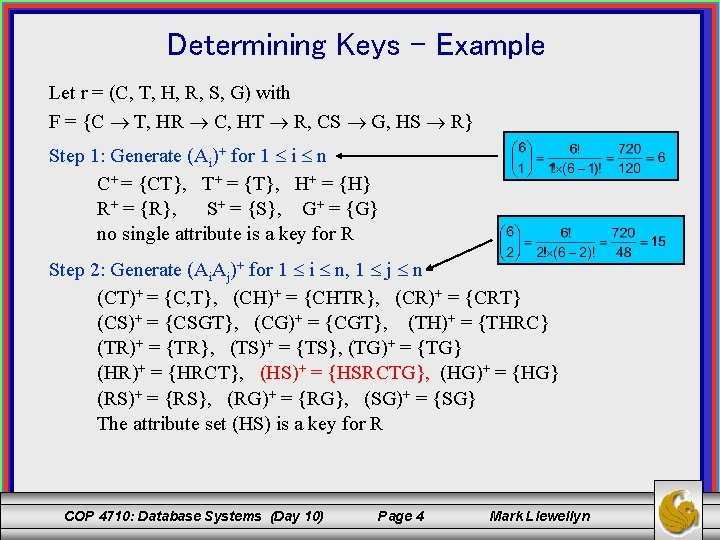Determining Keys - Example Let r = (C, T, H, R, S, G) with F = {C T, HR C, HT R, CS G, HS R} Step 1: Generate (Ai)+ for 1 i n C+ = {CT}, T+ = {T}, H+ = {H} R+ = {R}, S+ = {S}, G+ = {G} no single attribute is a key for R Step 2: Generate (Ai. Aj)+ for 1 i n, 1 j n (CT)+ = {C, T}, (CH)+ = {CHTR}, (CR)+ = {CRT} (CS)+ = {CSGT}, (CG)+ = {CGT}, (TH)+ = {THRC} (TR)+ = {TR}, (TS)+ = {TS}, (TG)+ = {TG} (HR)+ = {HRCT}, (HS)+ = {HSRCTG}, (HG)+ = {HG} (RS)+ = {RS}, (RG)+ = {RG}, (SG)+ = {SG} The attribute set (HS) is a key for R COP 4710: Database Systems (Day 10) Page 4 Mark Llewellyn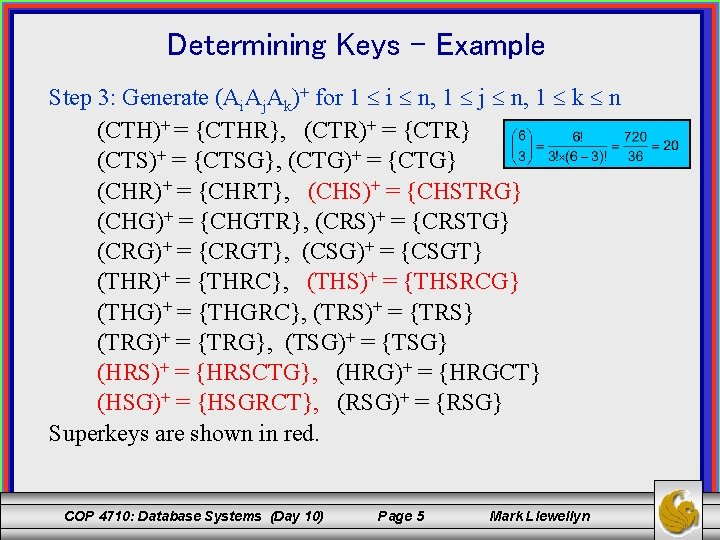Determining Keys - Example Step 3: Generate (Ai. Aj. Ak)+ for 1 i n, 1 j n, 1 k n (CTH)+ = {CTHR}, (CTR)+ = {CTR} (CTS)+ = {CTSG}, (CTG)+ = {CTG} (CHR)+ = {CHRT}, (CHS)+ = {CHSTRG} (CHG)+ = {CHGTR}, (CRS)+ = {CRSTG} (CRG)+ = {CRGT}, (CSG)+ = {CSGT} (THR)+ = {THRC}, (THS)+ = {THSRCG} (THG)+ = {THGRC}, (TRS)+ = {TRS} (TRG)+ = {TRG}, (TSG)+ = {TSG} (HRS)+ = {HRSCTG}, (HRG)+ = {HRGCT} (HSG)+ = {HSGRCT}, (RSG)+ = {RSG} Superkeys are shown in red. COP 4710: Database Systems (Day 10) Page 5 Mark Llewellyn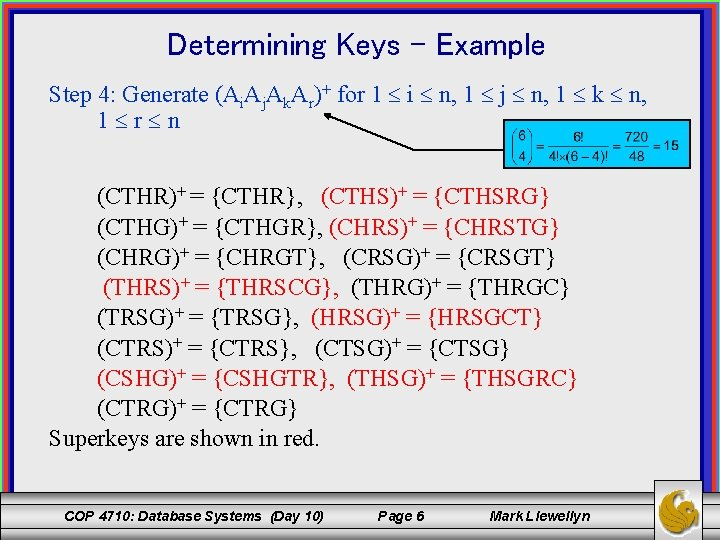Determining Keys - Example Step 4: Generate (Ai. Aj. Ak. Ar)+ for 1 i n, 1 j n, 1 k n, 1 r n (CTHR)+ = {CTHR}, (CTHS)+ = {CTHSRG} (CTHG)+ = {CTHGR}, (CHRS)+ = {CHRSTG} (CHRG)+ = {CHRGT}, (CRSG)+ = {CRSGT} (THRS)+ = {THRSCG}, (THRG)+ = {THRGC} (TRSG)+ = {TRSG}, (HRSG)+ = {HRSGCT} (CTRS)+ = {CTRS}, (CTSG)+ = {CTSG} (CSHG)+ = {CSHGTR}, (THSG)+ = {THSGRC} (CTRG)+ = {CTRG} Superkeys are shown in red. COP 4710: Database Systems (Day 10) Page 6 Mark Llewellyn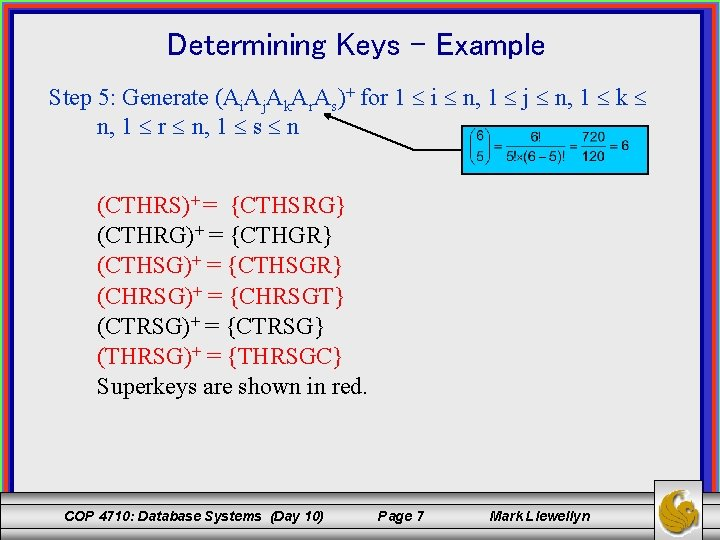Determining Keys - Example Step 5: Generate (Ai. Aj. Ak. Ar. As)+ for 1 i n, 1 j n, 1 k n, 1 r n, 1 s n (CTHRS)+ = {CTHSRG} (CTHRG)+ = {CTHGR} (CTHSG)+ = {CTHSGR} (CHRSG)+ = {CHRSGT} (CTRSG)+ = {CTRSG} (THRSG)+ = {THRSGC} Superkeys are shown in red. COP 4710: Database Systems (Day 10) Page 7 Mark Llewellyn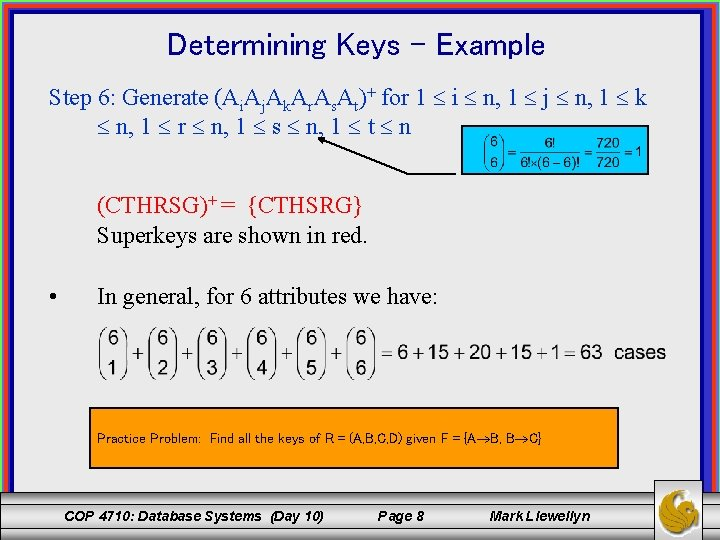Determining Keys - Example Step 6: Generate (Ai. Aj. Ak. Ar. As. At)+ for 1 i n, 1 j n, 1 k n, 1 r n, 1 s n, 1 t n (CTHRSG)+ = {CTHSRG} Superkeys are shown in red. • In general, for 6 attributes we have: Practice Problem: Find all the keys of R = (A, B, C, D) given F = {A B, B C} COP 4710: Database Systems (Day 10) Page 8 Mark Llewellyn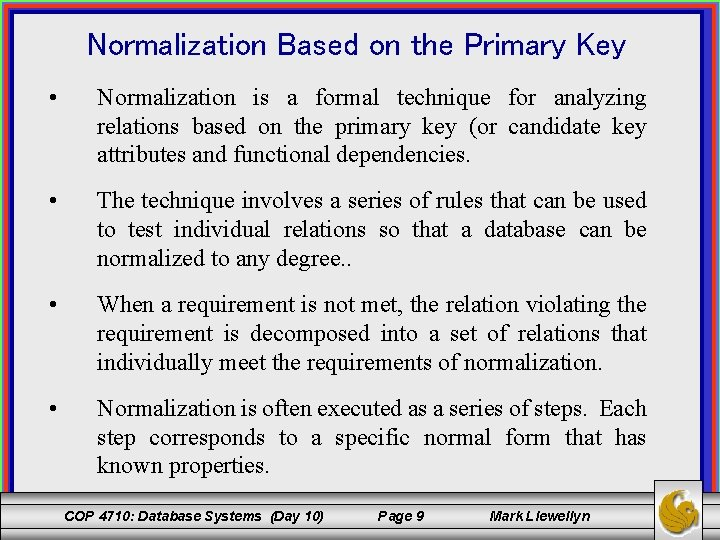Normalization Based on the Primary Key • Normalization is a formal technique for analyzing relations based on the primary key (or candidate key attributes and functional dependencies. • The technique involves a series of rules that can be used to test individual relations so that a database can be normalized to any degree. . • When a requirement is not met, the relation violating the requirement is decomposed into a set of relations that individually meet the requirements of normalization. • Normalization is often executed as a series of steps. Each step corresponds to a specific normal form that has known properties. COP 4710: Database Systems (Day 10) Page 9 Mark Llewellyn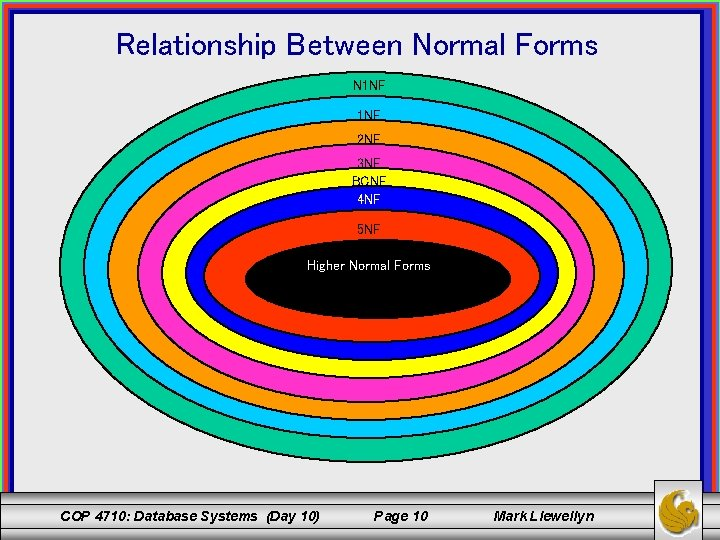Relationship Between Normal Forms N 1 NF 2 NF 3 NF BCNF 4 NF 5 NF Higher Normal Forms COP 4710: Database Systems (Day 10) Page 10 Mark LlewellynNormalization Requirements • For the relational model it is important to recognize that it is only first normal form (1 NF) that is critical in creating relations. All the subsequent normal forms are optional. • However, to avoid the update anomalies that we discussed earlier, it is normally recommended that the database designer proceed to at least 3 NF. • As the figure on the previous page illustrates, some 1 NF relations are also in 2 NF and some 2 NF relations are also in 3 NF, and so on. • As we proceed, we’ll look at the requirements for each normal form and a decomposition technique to achieve relation schemas in that normal form. COP 4710: Database Systems (Day 10) Page 11 Mark Llewellyn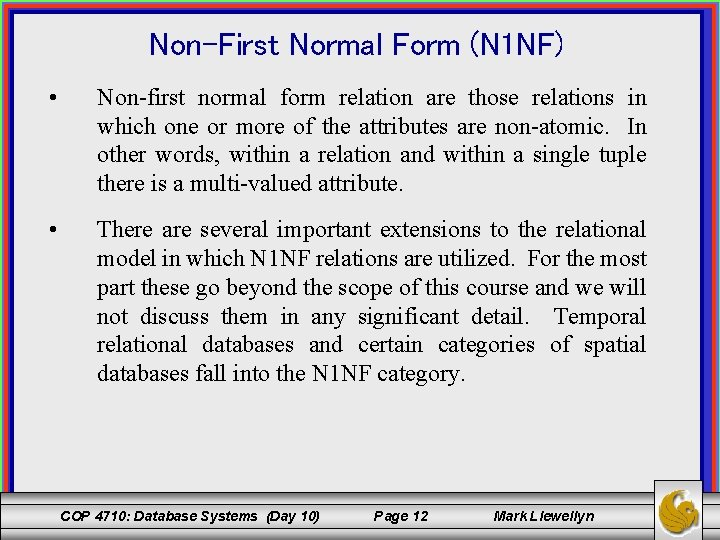Non-First Normal Form (N 1 NF) • Non-first normal form relation are those relations in which one or more of the attributes are non-atomic. In other words, within a relation and within a single tuple there is a multi-valued attribute. • There are several important extensions to the relational model in which N 1 NF relations are utilized. For the most part these go beyond the scope of this course and we will not discuss them in any significant detail. Temporal relational databases and certain categories of spatial databases fall into the N 1 NF category. COP 4710: Database Systems (Day 10) Page 12 Mark LlewellynFirst Normal Form (1 NF) • A relation in which every attribute value is atomic is in 1 NF. • We have only considered 1 NF relations for the most part in this course. • When dealing with multi-valued attributes at the conceptual level, recall that in the conversion into the relational model created a separate table for the multivalued attribute. (See Day 6, Pages 8 -10) COP 4710: Database Systems (Day 10) Page 13 Mark LlewellynSome Additional Terminology • A key is a superkey with the additional property that the removal of any attribute from the key will cause it to no longer be a superkey. In other words, the key is minimal in the number of attributes. • The candidate key for a relation a set of minimal keys of the relation schema. • The primary key for a relation is a selected candidate key. All of the remaining candidate keys (if any) become secondary keys. • A prime attribute is any attribute of the schema of a relation R that is a member of any candidate key of R. • A non-prime attribute is any attribute of R which is not a member of any candidate key. COP 4710: Database Systems (Day 10) Page 14 Mark Llewellyn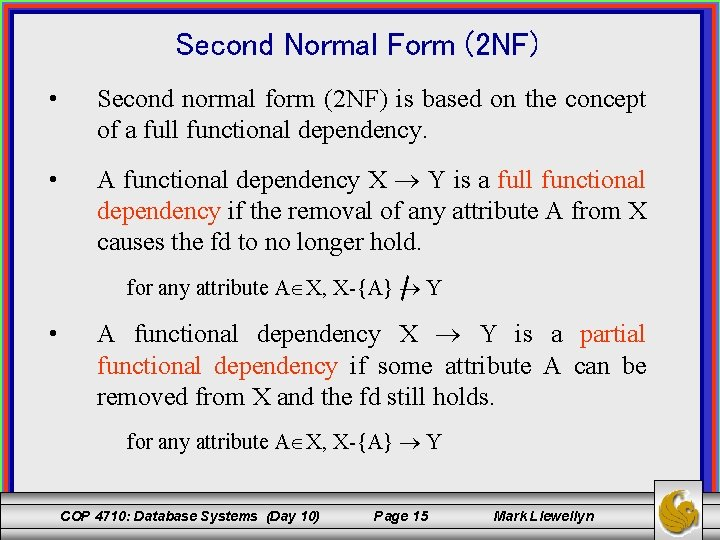Second Normal Form (2 NF) • Second normal form (2 NF) is based on the concept of a full functional dependency. • A functional dependency X Y is a full functional dependency if the removal of any attribute A from X causes the fd to no longer hold. for any attribute A X, X-{A} Y • A functional dependency X Y is a partial functional dependency if some attribute A can be removed from X and the fd still holds. for any attribute A X, X-{A} Y COP 4710: Database Systems (Day 10) Page 15 Mark Llewellyn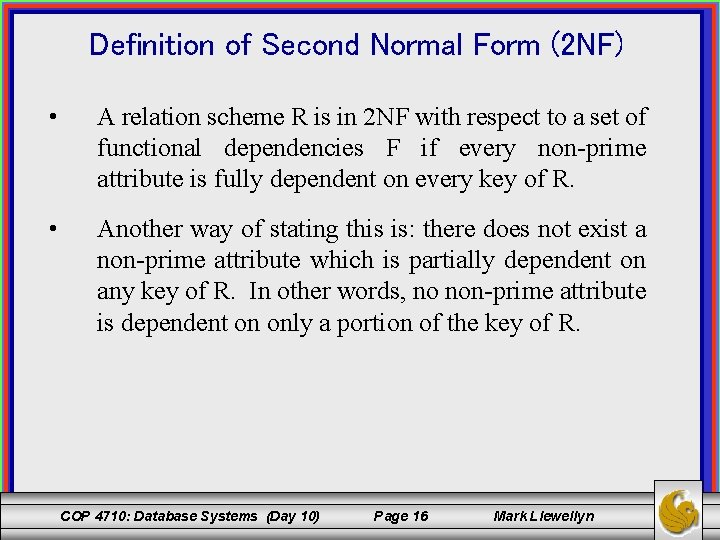Definition of Second Normal Form (2 NF) • A relation scheme R is in 2 NF with respect to a set of functional dependencies F if every non-prime attribute is fully dependent on every key of R. • Another way of stating this is: there does not exist a non-prime attribute which is partially dependent on any key of R. In other words, no non-prime attribute is dependent on only a portion of the key of R. COP 4710: Database Systems (Day 10) Page 16 Mark LlewellynExample of Second Normal Form (2 NF) Given R = (A, D, P, G), F = {AD PG, A G} and K = {AD} Then R is not in 2 NF because G is partially dependent on the key AD since AD G yet A G. Decompose R into: R 1 = (A, D, P) R 2 = (A, G) K 1 = {AD} K 2 = {A} F 1 = {AD P} F 2 = {A G} COP 4710: Database Systems (Day 10) Page 17 Mark Llewellyn